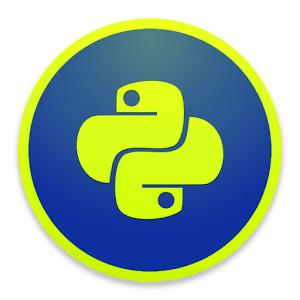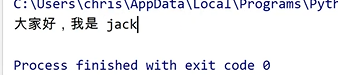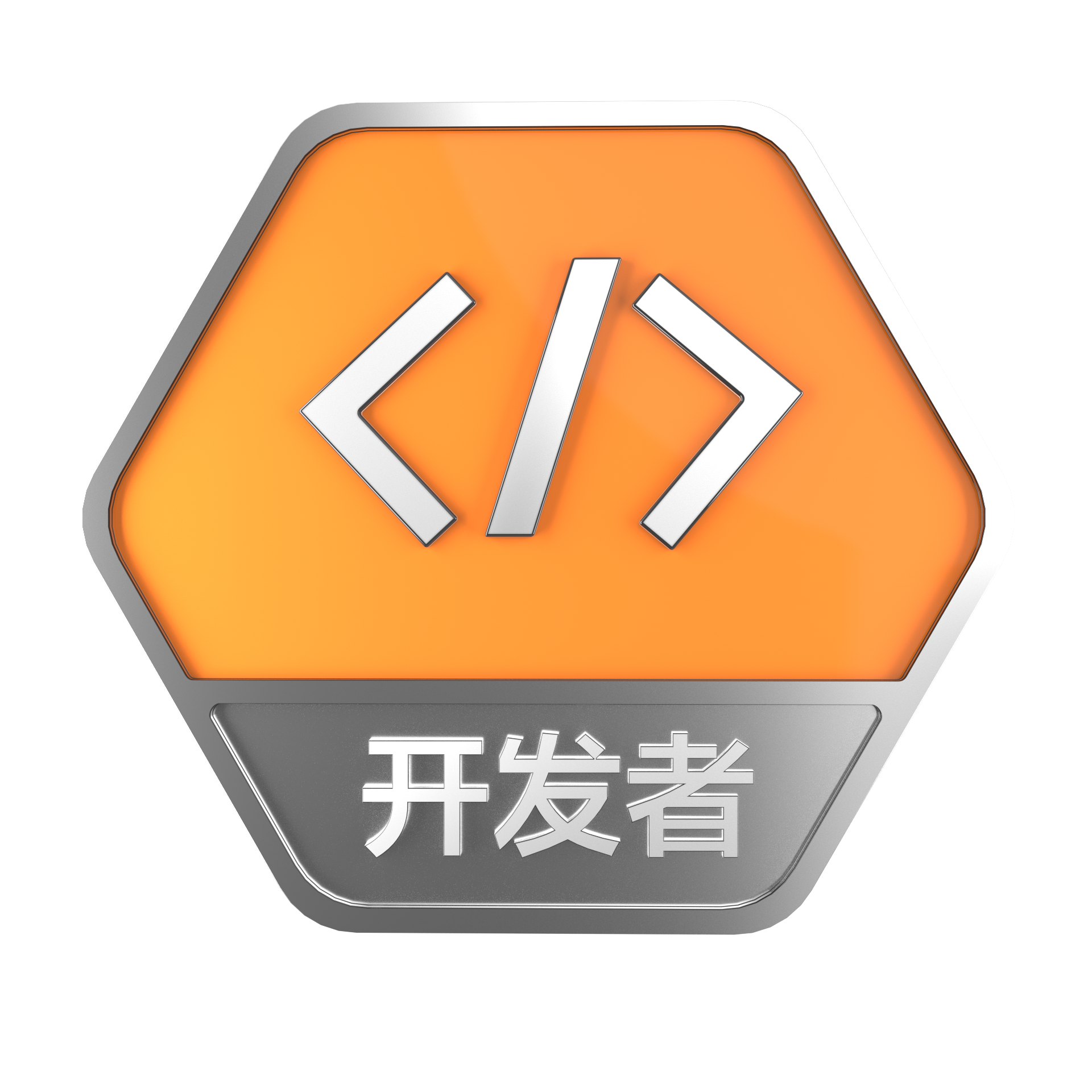# 进程池的使用 | 手把手教你入门Python之一百零九

+关注继续查看

## 进程池的使用

### Pool

def task(n):
print('{}----->start'.format(n))
time.sleep(1)
print('{}------>end'.format(n))

if __name__ == '__main__':
p = Pool(8)  # 创建进程池，并指定线程池的个数，默认是CPU的核数
for i in range(1, 11):
p.close()
p.join()

def task(n):
print('{}----->start'.format(n))
time.sleep(1)
print('{}------>end'.format(n))
return n ** 2

if __name__ == '__main__':
p = Pool(4)
for i in range(1, 11):
res = p.apply_async(task, args=(i,))  # res 是任务的执行结果
print(res.get())  # 直接获取结果的弊端是，多任务又变成同步的了
p.close()
# p.join()  不需要再join了，因为 res.get()本身就是一个阻塞方法

import time
from multiprocessing.pool import Pool

print('{}----->start'.format(n))
time.sleep(1)
print('{}------>end'.format(n))
return n ** 2

if __name__ == '__main__':
p = Pool(4)
res_list = []
for i in range(1, 11):
res_list.append(res)  # 使用列表来保存进程执行结果
for re in res_list:
print(re.get())
p.close()

from multiprocessing import Pool
import os, time, random

def worker(msg):
t_start = time.time()
print("%s开始执行,进程号为%d" % (msg, os.getpid()))
# random.random()随机生成0~1之间的浮点数
time.sleep(random.random() * 2)
t_stop = time.time()
print(msg, "执行完毕，耗时%0.2f" % (t_stop - t_start))

if __name__ == '__main__':
po = Pool(3)  # 定义一个进程池，最大进程数3
for i in range(0, 10):
# Pool().apply_async(要调用的目标,(传递给目标的参数元祖,))
# 每次循环将会用空闲出来的子进程去调用目标
po.apply_async(worker, (i,))

print("----start----")
po.close()  # 关闭进程池，关闭后po不再接收新的请求
po.join()  # 等待po中所有子进程执行完成，必须放在close语句之后
print("-----end-----")

----start----
0开始执行,进程号为21466
1开始执行,进程号为21468
2开始执行,进程号为21467
0 执行完毕，耗时1.01
3开始执行,进程号为21466
2 执行完毕，耗时1.24
4开始执行,进程号为21467
3 执行完毕，耗时0.56
5开始执行,进程号为21466
1 执行完毕，耗时1.68
6开始执行,进程号为21468
4 执行完毕，耗时0.67
7开始执行,进程号为21467
5 执行完毕，耗时0.83
8开始执行,进程号为21466
6 执行完毕，耗时0.75
9开始执行,进程号为21468
7 执行完毕，耗时1.03
8 执行完毕，耗时1.05
9 执行完毕，耗时1.69
-----end-----

multiprocessing.Pool常用函数解析：

• apply_async(func[, args[, kwds]]) ：使用非阻塞方式调用func（并行执行，堵塞方式必须等待上一个进程退出才能执行下一个进程），args为传递给func的参数列表，kwds为传递给func的关键字参数列表；
• close()：关闭Pool，使其不再接受新的任务；
• terminate()：不管任务是否完成，立即终止；
• join()：主进程阻塞，等待子进程的退出， 必须在close或terminate之后使用；

### 进程池中的Queue

RuntimeError: Queue objects should only be shared between processes through inheritance.

# 修改import中的Queue为Manager
from multiprocessing import Manager, Pool
import os, time, random

for i in range(q.qsize()):

def writer(q):
print("writer启动(%s),父进程为(%s)" % (os.getpid(), os.getppid()))
for i in "helloworld":
q.put(i)

if __name__ == "__main__":
print("(%s) start" % os.getpid())
q = Manager().Queue()  # 使用Manager中的Queue
po = Pool()
po.apply_async(writer, (q,))

time.sleep(1)  # 先让上面的任务向Queue存入数据，然后再让下面的任务开始从中取数据

po.close()
po.join()
print("(%s) End" % os.getpid())

(4171) start
writer启动(4173),父进程为(4171)
(4171) End

### join方法的使用

# join 线程和进程都有join方法
import time

x = 10

def test(a, b):
time.sleep(1)
global x
x = a + b

# test(1, 1)
# print(x)  # 2

t.start()
t.join()  # 让主线程等待

print(x)  # 10

### 配套视频1545 0<div class="markdown_views"> <p></p> <div class="toc"> <div class="toc"> <ul> <li><a href="#%E4%B8%80-%E4%BA%86%E8%A7%A3%E8%BF%9B%E7%A8%8B%E8%B5%84%E6%BA%90%E5%9B%BE">一 了解进程资源图</a></li> <li>
1290 0886 0933 0IPython、Notebook、qtconsole使用教程
IPython、Notebook、qtconsole使用教程 上一篇为Python,IPython,qtconsole,Notebook,Jupyter快速安装教程 1. 使用IPython 自动补全功能，使用tab键，如输入im后按tab键，可自动补全import。 进入IPython，CMD中输入ipython 退出IPython，CMD中输入quit()
3690 01669 07663 0self的使用 | 手把手教你入门Python之五十二

861 0【资料下载】Python 第七讲——从实战中了解异步爬虫aiohttp的使用

1839 0WPF 使用RPC调用其他进程

644 0+关注
1172

2

Python学习资料大全，包含Python编程学习、实战案例分享、开发者必知词条等内容。
+ 订阅

《SaaS模式云原生数据仓库应用场景实践》

《看见新力量：二》电子书